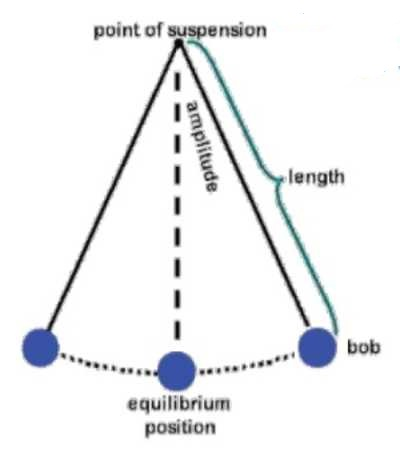# First Law of Simple Pendulum – Law of Isochronisms

First Law of Simple Pendulum—Law of isochronisms: When the angular amplitude of oscillation does not exceed 4°, the time period of oscillation of a simple pendulum of fix length at a given place is always constant, being independent of amplitude.

This law tells us that if θ ≤ 4° and g and L remain constant, then time period, T = constant for each oscillation. In 1582 Galileo discovered this law.Explanation

The first law of the simple pendulum states that the period of oscillation of a simple pendulum of constant length is independent of its amplitude provided the amplitude is small. Let us conduct an activity to verify the first law of the simple pendulum. To verify the first law, the apparatus required are a metallic bob, a thin and strong thread of length around 120 cm, an iron stand with a clamp, a cork vertically cut into two parts, a metre scale and a stop watch. Place the iron stand over a table. Tie the metallic bob to the thread and suspend it from the stand with the help of the cork and clamp. The simple pendulum is set up.

Choose a length for the pendulum, say 50 cm. Pull the bob to a side such that the amplitude is about 10 cm and allow the pendulum to oscillate. When the bob is at one of the extreme positions, start the stop watch. Count 20 oscillations and stop the stop watch. Note the time taken for 20 oscillations. Calculate the time taken for one oscillation, which is the time period of oscillation. Now repeat the procedure with amplitudes 15 cm and 20 cm. don’t change the length of the pendulum. Then tabulate the readings. We will observe that the time period of oscillation remains the same for different amplitudes. Thus, the period of oscillation is independent of amplitude, provided the amplitudes are small. This verifies the first law.